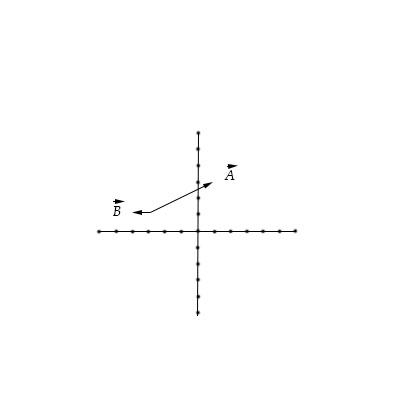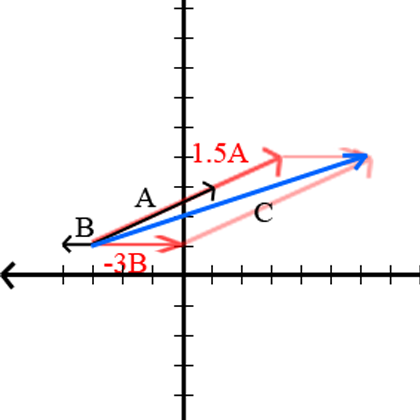# Draw the vector \vec C = 1.5 \vec A-3 \vec B.

## Question:

Draw the vector {eq}\vec C = 1.5 \vec A-3 \vec B. {/eq}## Vector Operations

Vectors are tools used to represent values with magnitude and direction. In graphs, these are arrows like in the picture above. Adding vectors is used to calculate the sum of vectors. As a basic example, a guy pushing a box will yield a force to where he is pushing it, a vector. Consequently, the ground will create friction opposite to the force he is pushing in, another vector but opposite. Taking the vector sum will show how fast the block accelerates with the man pushing.

From the graph, it is visible that {eq}\vec{A} {/eq} has 4 units to the right, and 2 units upward. Thus,

{eq}\vec{A} = 4\hat{i} + 2\hat{j} {/eq} and

B has one unit to the left. Thus,

{eq}\vec{B} = -\hat{i} {/eq}

Now, to get {eq}\vec{C} {/eq} , and by scalar multiplication

{eq}\begin{align} \Rightarrow 1.5\vec{A} &= 6\hat{i} + 3\hat{j} \\ -3\vec{B} &= 3\hat{i} \\ \end{align} {/eq}

Then, \vec{C}

{eq}\begin{align} \Rightarrow \vec{C} &= 1.5\vec{A} - 3\vec{B} \\ &= 1.5\vec{A} + (-3)\vec{B} \\ &= 6\hat{i} + 3\hat{j} + 3\hat{i} \\ &= 9\hat{i} + 3\hat{j} \\ \end{align} {/eq}

Thus,